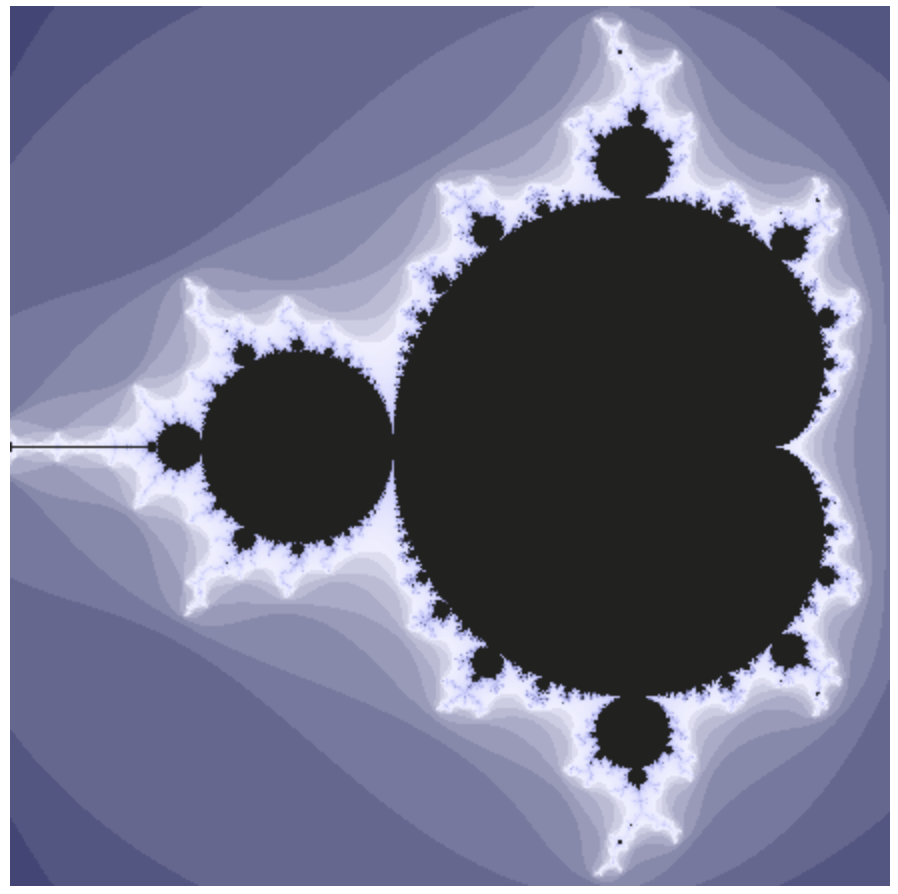# Understanding the Mandelbrot Fractal

By Paul Butler – August 15, 2018

The Mandelbrot fractal is a shape with incredible visual complexity: as you enlarge it, new details emerge at every level. It's quite possible to explore areas of the fractal that nobody has ever seen before.Given this complexity, you might assume that the math is beyond your grasp. In fact, the image of a Mandelbrot fractal above was generated with just addition and multiplication. Let's start with a refresher on imaginary and complex numbers, and then build on that to understand where the Mandelbrot fractal comes from.

## Imaginary Numbers

One of the core tools in math is abstraction: the idea that we can take a symbol, like $x$, and use that as a placeholder for an unknown value.

For example, if I say $x^2 = 4$, you can figure out that either $x = 2$ or $x = -2$. If I say $y^2 = -4$, we know that there is no real value of $y$ that I could possibly fill in to satisfy this equation. But if we can use symbols to represent an unknown number, why not use symbols to represent an unknowable number?

It turns out that, at least in one case, this is a useful thing to do: the imaginary numbers arise from defining $i$ such that $i^2 = -1$, even though we can't solve $i$ in the traditional sense. $i$ is called the imaginary unit, and the remaining imaginary numbers are just multiples of $i$.

 -2i-1i01i2i -2i-1i01i2i $1.0i \times 1.0i = 1i^2 = -1$

Let's have a look at what happens when we multiply two imaginary numbers together. Click the sliders above to change the numbers. Notice how the $i$s from each side combine into $i^2$, which we can replace by $-1$ because we decided earlier that $i^2 = -1$.

## Complex Numbers

When we add normal numbers to imaginary numbers, we call the resulting numbers complex. These numbers have two parts: a real part and an imaginary part. We can represent complex numbers visually by extending the imaginary sliders above to have a horizontal component.

 $1 + 0i$ -2i-1i1i2i-2-1012

The above plot is interactive; you can change the number by clicking anywhere in the chart area. We'll see more of these, so practice using the selector by finding the number $0.5 - 0.1i$.

Adding complex numbers is straightforward: we add the real parts, and add the imaginary parts, but we keep them separate.

 $a = 1 + 1i$ $b = -1.5 + 0.4i$ $a + b = -0.5 + 1.4i$ -2i-1i1i2i-2-1012 -2i-1i1i2i-2-1012 -2i-1i1i2i-2-1012

Throughout this page, complex number plots shown in red are interactive. Click within the plot to change the value, and see how the other values (shown in blue) change.

### Complex Multiplication

Multiplication is a bit more tricky, but it's really just an application of the FOIL method followed by a simplification using the rule $i^2 = -1$.

For example, consider $(a + bi) \times (c + di)$. By FOIL we get $ac + adi + bci + bdi^2$. Replacing $i^2$ for $-1$, we can simplify this to $(ac - bd) + (ad + bc)i$.

 $a = 1 + 1i$ $b = -0.5 + 1.2i$ $a \times b = -1.7 + 0.7i$ -2i-1i1i2i-2-1012 -2i-1i1i2i-2-1012 -2i-1i1i2i-2-1012

Squaring a complex number is just a special case of multiplication: $(a + bi)^2 = (a^2 - b^2) + (2ab)i$.

## A Curious Pattern

It turns out that if we combine complex multiplication and addition in a certain way, an interesting pattern emerges. First, we start with any complex number $c$, and start with $z = 0 + 0i$. Then, we set $z \gets z^2 + c$ over and over again.

Try picking a value of $c$ below, and then hit the step button a bunch of times. Each time you hit step, the value of $z$ is set to $z^2 + c$.

For each value of $c$, one of two things will happen: either the point will fly out of the outer ring (maybe after thrashing around within it for a bit), or the point will settle into a pattern in which it will apparently never leave the ring.

 $c = 0.3 + 0.5i$ $z = 0 + 0i$ $z^2 = 0 + 0i$ $z^2 + c = 0.3 + 0.5i$ -2i-1i1i2i-2-1012 -2i-1i1i2i-2-1012 -2i-1i1i2i-2-1012 -2i-1i1i2i-2-1012

You might wonder whether there is any pattern to which values of $c$ cause $z$ to fly out of the ring instead of remaining inside it. Imagine placing a transparent grid on top of the $c$ picker. Then, you could methodically move $c$ to each point on the grid, hit step a bunch of times, and mark a dot over every $c$ value that did not fly off.

Of course, that would be a time-consuming exercise, but it essentially describes what happens when you brush over the image below. As you mouse over the grid, the plot on the right shows the progression of $z$ found when calculating the grid cell under your cursor. We color points according to how many steps $z$ remained inside the outer circle for before it escaped it, up to 200 steps.

 -2i-1i1i2i-2-1012

As you can see, as you mouse over the image, the Mandelbrot fractal reveals itself. Try double-clicking to zoom in.

I hope that the magic of the Mandelbrot fractal is not diminished by seeing how simple the math is, but instead that seeing such complexity arise from a simple rule gives you a new sense of appreciation for it.